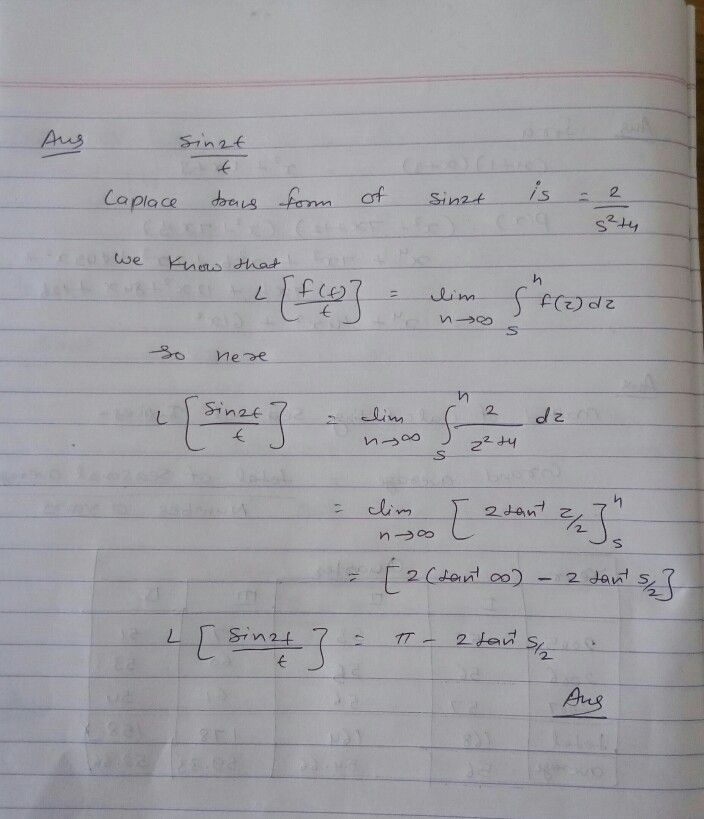Symbol
Problem$\dfrac {sin2} {t}$ $1\right)$ The Laplace transform $0f$ the function $is$ $5$
Other
Search count: 105
SolutionQanda teacher - VishalStudent
thank you